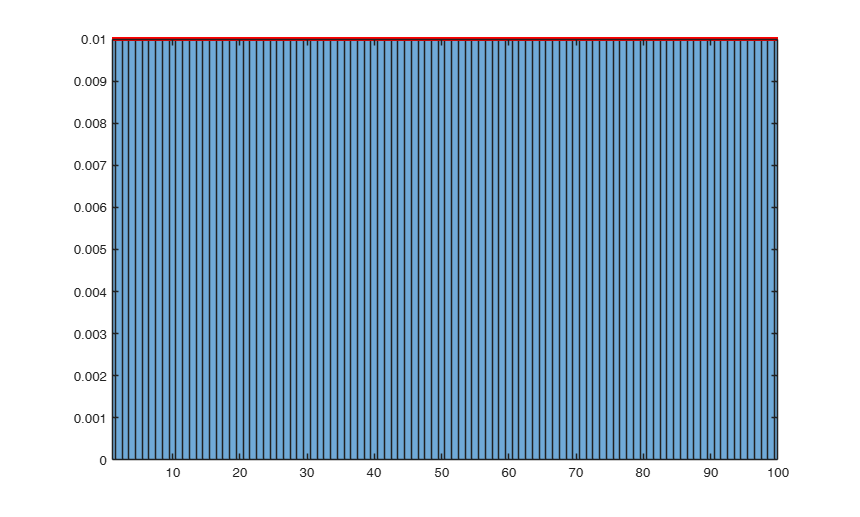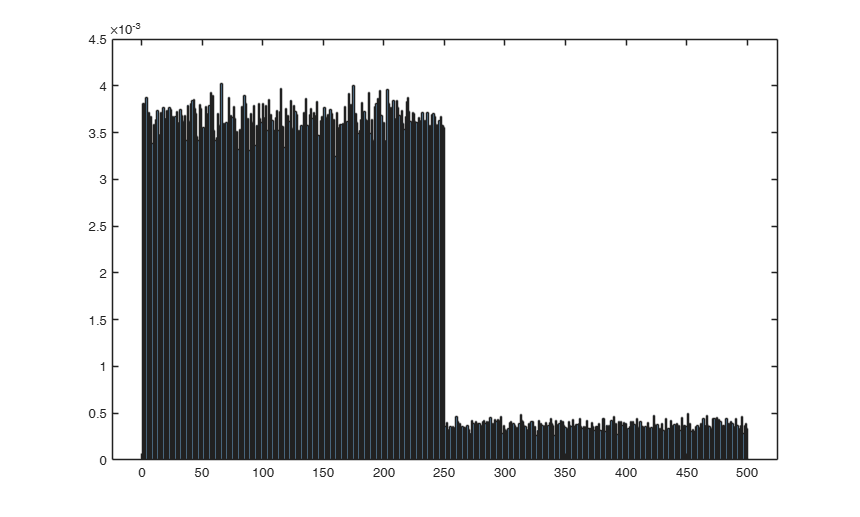# subsets

subsets creates a matrix of indexes where rows are distinct p-subsets extracted from a set of n elements

## Syntax

• C=subsets(nsamp, n, p)example
• C=subsets(nsamp, n, p, ncomb)example
• C=subsets(nsamp, n, p, ncomb, msg)example
• C=subsets(nsamp, n, p, ncomb, msg, method)example
• [C,nselected]=subsets(___)example

## Description

 C =subsets(nsamp, n, p) Create a matrix with the indexes of 5 subsets when n=100, p=3.

 C =subsets(nsamp, n, p, ncomb) Create a matrix with the indexes of 5 subsets when n=100, p=3.

 C =subsets(nsamp, n, p, ncomb, msg) Create a matrix with the indexes of 5 subsets when n=100, p=3.

 C =subsets(nsamp, n, p, ncomb, msg, method) Create a matrix with the indexes of 5 subsets when n=100, p=3.

 [C, nselected] =subsets(___) Extract 80000 subsets and check they are unique.

## Examples

expand all

###Create a matrix with the indexes of 5 subsets when n=100, p=3.

Only default arguments used.

C = subsets(5,100,3)
C =

5×3 int8 matrix

30   41   85
19   84   95
7   59   61
46   50   54
77   97   98



###Create a matrix with the indexes of 5 subsets when n=100, p=3.

Use information on the number of combinations to speed up generation.

ncomb = bc(100,3);
C = subsets(5,100,3,ncomb)
C =

5×3 int8 matrix

19   20   70
10   55   88
13   53   67
5   46   74
52   59   67



###Create a matrix with the indexes of 5 subsets when n=100, p=3.

Also inform about the time taken for the operation.

ncomb = bc(1000,5);
C = subsets(500000,1000,5,ncomb,1);
Warning: you have specified to extract more than 100000 subsets
To iterate so many times may be lengthy


### Create a matrix with the indexes of 5 subsets when n=100, p=3.

As the previous example, but in addition returns in nselected the number of combinations.

ncomb = bc(1000,5);
[C , nselected] = subsets(500000,1000,5,ncomb,1);

### Extract 80000 subsets and check they are unique.

C=subsets(80000,100,5);
size(unique(C,'rows'))

## Related Examples

expand all

###Sampling without replacement and the hypergeometric distribution.

clear all; close all;
% parameters
n      = 100;
p      = 3;
nsamp  = 1000000;
ncomb  = bc(n,p);
msg    = 0;
% Sampling without repetition nsamp p-subsets from a dataset of n units.
C = subsets(nsamp, n, p, ncomb, msg);
if verLessThan('matlab','8.4')
hist(double(C(:))); xlim([1 n]);
else
histogram(double(C(:)),'Normalization','pdf','BinMethod','Integers'); xlim([1 n]);
% this superimposes a line with the unit counts
frC = tabulateFS(double(C(:)));
hold on; plot(1:n,frC(:,3)/100,'r-','LineWidth',3);
end
% The hypergeometric distribution hygepdf(X,M,K,N) computes the probability
% of drawing exactly X of a possible K items in N drawings without
% replacement from a group of M objects. For drawings with replacement,
% the distribution would be binomial.
hpdf = hygepdf(0:p,n,n/2,p);
% Say that the n/2 target items (which determine the success of a draw) are
% in the subset formed by units 1,2,...n/2. Let's then count how many times
% we get units from this group.
c   = C<=n/2;
sc  = sum(c,2);
tab = tabulateFS(sc);
tab = (tab(:,2)/sum(tab(:,2)))';
disp('Probability of getting 0 to p successes in p drawns (hypergeometric pdf):');
disp(hpdf);
disp('Frequencies of the 0 to p successes in the p drawns (subsets output):');
disp(tab);
Warning: number of subsets which have been chosen (1000000) are greater than possibile number (161700)
All subsets are extracted
Probability of getting 0 to p successes in p drawns (hypergeometric pdf):
0.1212    0.3788    0.3788    0.1212

Frequencies of the 0 to p successes in the p drawns (subsets output):
0.1212    0.3788    0.3788    0.1212###Weighted sampling without replacement and the non-central Wallenius hypergeometric distribution.

clear all; close all;
% parameters
n      = 500;
p      = 3;
nsamp  = 50000;
ncomb  = bc(n,p);
msg    = 0;
% Sampling probability of the first n/2 units is 10 times larger than the others n/2.
method = [10*ones(n/2,1); ones(n/2,1)];
% no need to normalize weights: method = method(:)' / sum(method);
C = subsets(nsamp, n, p, ncomb, msg, method);
if verLessThan('matlab','8.4')
hist(double(C(:))); xlim([1 n]);
else
histogram(double(C(:)),'Normalization','pdf','BinMethod','Integers');
end
% Here we address the case when the sampling (without replacement) is biased,
% in the sense that the probabilities to select the units in the sample are
% proportional to weights provided using option 'method'. In this case, the
% extraction probabilities follow Wallenius' noncentral hypergeometric
% distribution. The sampling scheme is the same of that of the hypergeometric
% distribution but, in addition, the success and failure are associated with
% weights w1 and w2 and we will say that the odds ratio is W = w1 / w2. The
% function is then called as: wpdf = WNChygepdf(x,N,K,M,W).
for i = 0:p
wpdf(i+1) = WNChygepdf(i,p,n/2,n,10);
end
% counts of the actual samples
c   = C<=n/2;
sc  = sum(c,2);
tab = tabulateFS(sc);
tab = (tab(:,2)/sum(tab(:,2)))';
disp('Probability of getting 0 to p successes in p weighted drawns (non-central hypergeometric pdf):');
disp(wpdf);
disp('Frequencies of the 0 to p successes in the p weighted drawns (subsets output):');
disp(tab);
% The non-central hypergeometric is also available in the R package
% BiasedUrn. In the example above, where there are just two groups and one
% weight defining the ratio between the units in the two groups, the function
% to use is dWNCHypergeo (for Wallenius' distribution):
%
% dWNCHypergeo(c(0,1,2,3), 50, 50, 3, 10)
%  0.0007107089 0.0225823308 0.2296133830 0.7470935773
%
% The general syntax of the function is:
% dWNCHypergeo(x, m1, m2, n, odds)
% x  = Number of red balls sampled.
% m1 = Initial number of red balls in the urn.
% m2 = Initial number of white balls in the urn.
% n  = Total number of balls sampled.
% N  = Total number of balls in urn before sampling.
% odds = Probability ratio of red over white balls.
% p = Cumulative probability.
% nran = Number of random variates to generate.
% mu = Mean x.
% precision = Desired precision of calculation.
Probability of getting 0 to p successes in p weighted drawns (non-central hypergeometric pdf):
0.0007    0.0225    0.2262    0.7505

Frequencies of the 0 to p successes in the p weighted drawns (subsets output):
0.0008    0.0221    0.2340    0.7431### Weighted sampling without replacement, with negative weights.

clear all; close all;
n = 200;
p = 3;
nsamp = 10000;
ncomb = bc(n,p);
msg = 0;
method = [-4*ones(n/4,1); -2*ones(n/4,1) ; -1*ones(n/4,1); -4*ones(n/4,1)];
C = subsets(nsamp, n, p, ncomb, msg, method);
if verLessThan('matlab','8.4')
hist(double(C(:))); xlim([1 n]);
else
histogram(double(C(:)),'Normalization','pdf','BinMethod','Integers');
end

###Function subset used in clustering or mixture modeling simulations.

clear all; close all;
% parameters
n      = 100;       %number of units
p      = 2;         %number of variables
k      = 3;         %number of groups
nsamp  = 500;       %number of samples
ncomb  = bc(n,p);
msg    = 0;
% A dataset simulated using MixSim
rng(372,'twister');
Q=MixSimreg(k,p,'BarOmega',0.001);
[y,X,id]=simdatasetreg(n,Q.Pi,Q.Beta,Q.S,Q.Xdistrib);
% Some user-defined weights for weighted sampling, provided as a vector of "method" option.
method = [1*ones(n/2,1); ones(n/2,1)];
% C must be a nsamp-by-k*p matrix, to contain the extraction of nsamp p-combinations k times.
% This can be easily done as follows:
for i=1:k
Ck(:,(i-1)*p+1:i*p) = subsets(nsamp, n, p, ncomb, msg, method);
end
% Ck is then provided, e.g., to tclustreg as follows:
out=tclustreg(y,X,k,50,0.01,0.01,'nsamp',Ck);
Warning: columns 1  2 are constant and just col 1 has been kept!
ClaLik with untrimmed units selected using crisp criterion
Total estimated time to complete tclustreg:  1.58 seconds## Input Arguments

### nsamp — Number of subsamples which have to be extracted. Scalar; if nsamp=0 all subsets will be extracted; they will be (n choose p).

Data Types: single|double

### n — Number of observations of the dataset. Scalar.

Data Types: single|double

### p — Size of the subsets. Scalar.

In regression with p explanatory variable the size of the elmental subsets is p;

in multivariate analysis, in presence of v variables, the size of the elemental subsets is v+1.

Data Types: single|double

### ncomb — scalar (n choose p). If the user has already computed this value it can supply it directly, otherwise the program will calculate it automatically.

Example: C=subsets(20,10,3,120) 

Data Types: single|double

### msg — scalar which controls whether to display or not messages on the screen. If msg=true (default), messages are displayed on the screen about estimated time.

Example: C=subsets(20,10,3,120,0) 

Data Types: boolean

### method — Sampling methods. Scalar or vector.

Methods used to extract the subsets. See section 'More About' of function randsampleFS for details about the sampling methods. Default is method = 1.

- Scalar, from 0 to 3 determining the (random sample without replacement) method to be used.

- Vector of weights: in such a case, Weighted Sampling Without Replacement is applied using that vector of weights.

Example: randsampleFS(100,10,2) 

Data Types: single|double

## Output Arguments

### C —The indices of the subsets which need to be extracted.  Matrix with nselected rows and p columns (stored in int16 format)

Data Types - single|double

### nselected —Number of rows of matrix C. Scalar

Data Types - single|double

Wong, C.K. and M.C. Easton (1980) "An Efficient Method for Weighted Sampling Without Replacement", SIAM Journal of Computing, 9(1):111-113.